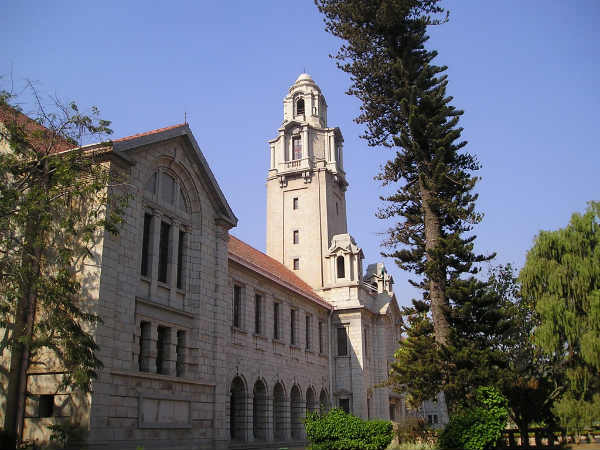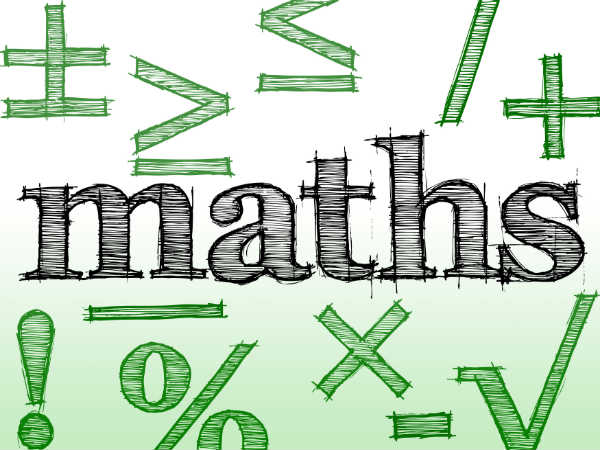# How Is Mathematics Used In Real Life

By Malavikka S

Mathematics is a subject that requires a lot of effort in terms of thinking, reasoning, understanding and solving. Usually, maths students are considered to be smart people because of the skills they possess.

In fact, during school, we have all studied maths every year, some times even cursing the complex calculations and procedures wondering what they have to do with our lives. Good news for you is that we have explored how maths and the real world are connected. Come, read on!

## Calculus

Calculus is a concept used to calculate the smallest difference in a number. It is divided into differentiation and integration. You might have studied the concept deeper in your school or college. But have you ever wondered what it actually means and what it is used for?

An architect uses calculus to calculate the materials required to construct curved structures such as domes and arches.

Electrical engineers use it to calculate the length of power cables between places that are miles apart.

Biologists use it to calculate the rate of growth in microbes.

## Algebra

"I know everybody's exes, other than algebra's 'x'." If that was one of your statements while you were wondering what that 'x' had to do in your life, then here's the answer to that burning question!

• The study of DNA and genes can be better explained in terms of equations. Their properties can be easily deduced with the help of x and y chromosomes.
• In economics, x and y are used to determine the supply and demand of goods.
• In computer graphics (CG), x and y are used as coordinates to design objects and their movements. The famous game Mario was designed using 3D animation on CG.

## Trigonometry

Sine, cos, tan and cosec. What were all those names anyway?! Each one denotes an angular measurement.

• Trigonometric angles are used in the construction of marine ramps.
• They are used to spot a location for navigation.
• They are also used to determine the depth of algae underwater and sonar systems.Top 10 Colleges In India For Maths In 2018

## Logarithms

We all can write numbers up to 10 million. Beyond that, can you read the numbers at one go without actually re-reading and counting the zeros? Logarithms simplify complicated numbers through exponents and coefficients. Log tables help you to refer the corresponding numbers and make calculations less laborious. It is largely used in computing memory spaces and in computer languages.

## Probability

Probability is a concept we use in everyday life. 'Should I take a car or bus to office?' is something we ask ourselves on a regular basis, and we make the decision using probability. Probability basically tells you the chances of a specific occurence of an event. The meticulous calculations can be used in determining big events such as the probability of you winning a jackpot or a lottery!

## Statistics

Statistics, like probability, is a more familiar concept. And like probability, we use statistics in our everyday lives. Population census is an epitome of statistics. To get the demographics of a sample size, statistics plays an important role with its various functions, like while determining average and mean estimates.7 Mathematics Shortcuts That Can Blow You Down

## Matrices

Matrix is an array of elements processed according to specific needs. In robotic systems, matrices are used to control the movement of robots. Data structures and databases make use of this concept for an organised algorithm.

## Mensuration

Mensuration is nothing but measurement of different units. From buying groceries to checking our weight, we use mensuration day in and day out. From determining the body temperature using a thermometer and a barometer to determining the weather, mensuration plays an integral role.

Subscribe Now

--Or--
Select a Field of Study
Select a Course
Select UPSC Exam
Select IBPS Exam
Select Entrance Exam